# Matrix

## 厄米特矩阵

• 厄米特矩阵 $$A^H = A$$
• 实对称矩阵 $$A^T = A$$
• 反厄米特矩阵 $$A^H = -A$$
• 实反对称矩阵 $$A^T = -A$$

## 正规矩阵

$A^H A = A A^H$

• 酉矩阵 $$A^H A = A A^H = I$$
• 正交矩阵 $$A^T A = AA^T = I$$
• 特殊正交矩阵：$$\det(A) = +1$$，是一个旋转矩阵
• Givens矩阵：$$G(i,j,\theta)$$
• 瑕旋转矩阵：$$\det(A) = -1$$，瑕旋转是旋转加上镜射
• Householder矩阵：$$H = I - 2 uu^T$$

## 正定与半正定矩阵

$f = \boldsymbol{x}^T \boldsymbol{A} \boldsymbol{x} > 0$

### 直观理解

$$Y=MX$$，则 $$X^T Y > 0$$，所以

$cos(\theta) = \frac{X^T Y}{\|X\|\|Y\|} > 0$

### 判别对称矩阵A的正定性

• 求出A的所有特征值
• 若A的特征值均为正数，则A是正定的；若A的特征值均为负数，则A为负定的。
• 计算A的各阶顺序主子式
• 若A的各阶顺序主子式均大于零，则A是正定的；若A的各阶顺序主子式中，奇数阶主子式为负，偶数阶为正，则A为负定的。

## 伴随矩阵

### 余子式

A关于第i行第j列的余子式（记作 $$M_{ij}$$）是去掉A的第i行第j列之后得到的(n− 1)×(n− 1)矩阵的行列式。

### 代数余子式

A的 (代数)余子矩阵 是一个n×n的矩阵C，使得其第i行第j列的元素是A关于第i行第j列的代数余子式：

$\mathbf{C}_{i j}=(-1)^{i+j} M_{i j}$

A的 代数余子矩阵 的 转置矩阵，也就是说， A的伴随矩阵是一个n×n的矩阵（记作adj(A)），使得其第i 行第j 列的元素是A关于第j 行第i 列的代数余子式

$\mathbf{A}^* = \operatorname{adj}(\mathbf{A})=\mathbf{C}^{T}$

### 自伴随矩阵

$\mathbf{A}^* = \mathbf{A}$

### 可逆矩阵（非奇异矩阵）

$\mathbf{A}^{-1} = \frac{1}{|\mathbf{A}|} \mathbf{A}^*$

• 矩阵乘以可逆矩阵 秩不变，因为可逆矩阵可以表示为初等矩阵的乘积，而初等变换不改变矩阵的秩（左乘-行变换，右乘-列变换）

## 其他

• 对角阵：任意正规矩阵 都可以经过 正交变换 变成 对角矩阵

• 上（下）三角阵

# 矩阵变换

## 初等变换

• 初等变换只是不影响矩阵的秩，其他的特性都改变了。

• 对于计算矩阵的行列式，不能进行初等变换，但是可以做行列的进加减，不能乘以系数。

## Givens rotation（吉文斯旋转）

$G(i, j, \theta)=\left[\begin{array}{ccccccc} 1 & \cdots & 0 & \cdots & 0 & \cdots & 0 \\ \vdots & \ddots & \vdots & & \vdots & & \vdots \\ 0 & \cdots & c & \cdots & s & \cdots & 0 \\ \vdots & & \vdots & \ddots & \vdots & & \vdots \\ 0 & \cdots & -s & \cdots & c & \cdots & 0 \\ \vdots & & \vdots & & \vdots & \ddots & \vdots \\ 0 & \cdots & 0 & \cdots & 0 & \cdots & 1 \end{array}\right]$

$G \triangleq\left[\begin{array}{cc} \cos \phi & \sin \phi \\ -\sin \phi & \cos \phi \end{array}\right]$

$\left[\begin{array}{cc} c & -s \\ s & c \end{array}\right]\left[\begin{array}{l} a \\ b \end{array}\right]=\left[\begin{array}{l} r \\ 0 \end{array}\right]$

\begin{aligned} &r \leftarrow \sqrt{a^{2}+b^{2}}\\ &c \leftarrow a / r\\ &s \leftarrow-b / r \end{aligned}

• Givens 旋转在数值线性代数中主要的用途是在向量或矩阵中介入零。例如，这种效果可用于计算矩阵的 QR分解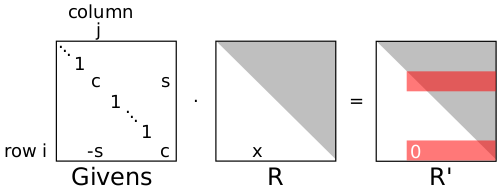$G_{j_{k}} \ldots G_{j_{2}} G_{j_{1}} R_{a}=\left[\begin{array}{c} R^{\prime} \\ 0 \end{array}\right]$

• 超过 Householder变换 的一个好处是它们可以轻易的并行化，另一个好处是对于非常稀疏的矩阵计算量更小。

## Householder 变换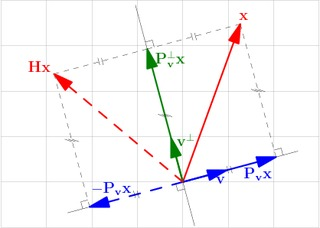$\mathbf{H}=\mathbf{I}-\frac{2}{\langle\mathbf{v}, \mathbf{v}\rangle} \mathbf{v} \mathbf{v}^{H}$

$\mathbf{v}=\mathbf{x}+\operatorname{sgn}\left(\mathbf{x}_{1}\right)\|\mathbf{x}\|_{2} \mathbf{e}_{1}$

# Matrix Decomposition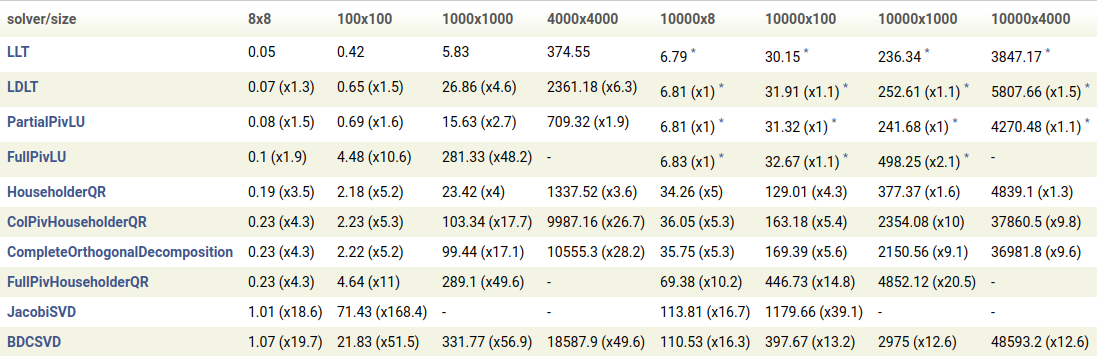## EVD (Eigen Decomposition)

$A = VDV^{-1}$

• $$A$$方阵$$D$$对角阵，其 特征值从大到小排列$$V$$ 的列向量为 特征向量
• $$A$$对称阵，则 $$V$$正交矩阵，其列向量为 $$A$$单位正交特征向量

## SVD (Singular Value Decomposition)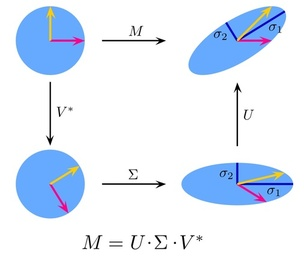$A = UDV^T$

• $$A$$$$m \times n$$ 的矩阵；$$D$$非负对角阵，其 奇异值从大到小排列$$U$$$$V$$ 均为 正交矩阵

SVD分解十分强大且适用，因为任意一个矩阵都可以实现SVD分解，而特征值分解只能应用于方阵。

### 奇异值与特征值

$AV = UD \Rightarrow Av_i = \sigma_{i} u_i \Rightarrow \sigma_{i} = \frac{Av_i}{u_i}$

$A^T A = (V D^T U^T) (U D V^T) = V D^2 V^T$

$A A^T = (U D V^T) (V D^T U^T) = U D^2 U^T$

• $$A^T A$$特征向量 组成的是SVD中的 $$V$$ 矩阵
• $$A A^T$$特征向量 组成的是SVD中的 $$U$$ 矩阵
• $$A^T A$$$$A A^T$$特征值 $$\lambda_i$$$$A$$奇异值 $$\sigma_i$$ 满足 $$\sigma_i = \sqrt{\lambda_i}$$

### PCA

$A_{m \times n} = U_{m \times m} D_{m \times n} V_{n \times n}^T \approx U_{m \times k} D_{k \times k} V_{k \times n}^T$

## LU Decomposition

$A = LU$

• $$A$$方阵$$L$$下三角矩阵$$U$$上三角矩阵

### PLU 分解

$A = PLU$

### LDU 分解

$A = LDU$

## Cholesky Decomposition

$A = LDL^T$

• $$A$$方阵正定矩阵$$L$$下三角矩阵

classic:

$A = LL^T \\[2ex] A^{-1} = (L^T)^{-1} L^{-1} = (L^{-1})^T L^{-1}$

## QR Decomposition

$A = QR$

• $$A$$$$m \times n$$ 的矩阵；$$Q$$酉矩阵$$R$$上三角矩阵

# Four Fundamental Subspaces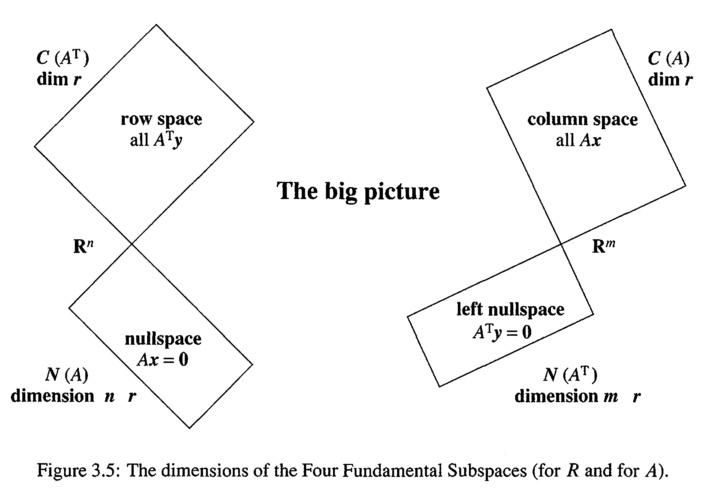• 零空间 与 行空间 正交
• 左零空间 与 列空间 正交

## column space (the range or image) $$C(A)$$

In linear algebra, the column space (also called the range or image) of a matrix A is the span (set of all possible linear combinations) of its column vectors. The column space of a matrix is the image or range of the corresponding matrix transformation.

## nullspace $$N(A)$$

$A x = 0$

The nullity of a matrix is the dimension of the null space.

The rank and nullity of a matrix A with n columns are related by the equation:

$\operatorname{rank}(A) + \operatorname{nullity}(A) = n$

## left nullspace $$N(A^T)$$

$A^T x = 0 \longrightarrow x^T A = 0$

### 左零空间 求解

#### SVD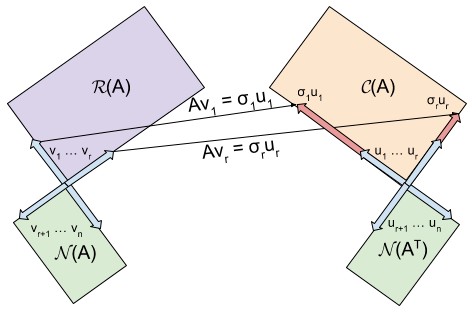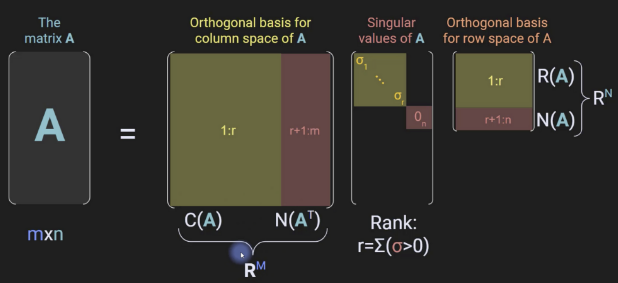\begin{aligned} A_{m \times n} &= U_{m \times m} \cdot {\Sigma}_{m \times n} \cdot V_{n \times n}^T \\ &= \left[\begin{array}{ccc|ccc} u_{1} & \cdots & u_{r} & u_{r+1} & \cdots & u_{m} \end{array}\right] \left[\begin{array}{ccc|c} \sigma_{1} & & & \\ & \ddots & & 0 \\ & & \sigma_{r} & \\ \hline & 0 & & 0 \end{array}\right] \left[\begin{array}{c} v_{1}^{T} \\ \vdots \\ v_{r}^{T} \\ \hline v_{r+1}^{T} \\ \vdots \\ v_{n}^{T} \end{array}\right] \\ &= \begin{bmatrix} {U_1}_{m \times r} & {U_2}_{m \times (m-r)} \end{bmatrix} \cdot \begin{bmatrix} {\Sigma}_r & 0 \\ 0 & 0 \end{bmatrix} \cdot \begin{bmatrix} {V_1}_{n \times r}^T \\[3pt] {V_2}_{n \times (n-r)}^T \end{bmatrix} \\ &= U_1 \cdot \Sigma_r \cdot V_1^T \\ &= \sum_{i=1}^r \sigma_i u_i v_i^T \end{aligned}

nullspace

$A \cdot V_2 = 0 \longrightarrow N(A) = V_2 \in \mathbb{R}^{n \times (n-r)}$

left nullspace

$U_2^T \cdot A = 0 \longrightarrow N(A^T) = U_2 \in \mathbb{R}^{(m-r) \times m}$

column space or the range (????)

$C(A) = U_1^T \in \mathbb{R}^{r \times m}$

#### QR

\begin{aligned} A_{m \times n} &= Q_{m \times m} \cdot R_{m \times n} \\ &= \begin{bmatrix} {Q_1}_{m \times r} & {Q_2}_{m \times (m-r)} \end{bmatrix} \begin{bmatrix} R_1 \\ 0 \end{bmatrix} \end{aligned}

left nullspace

$Q_2^T \cdot A = 0 \longrightarrow N(A^T) = Q_2 \in \mathbb{R}^{(m-r) \times m}$

# 线性方程组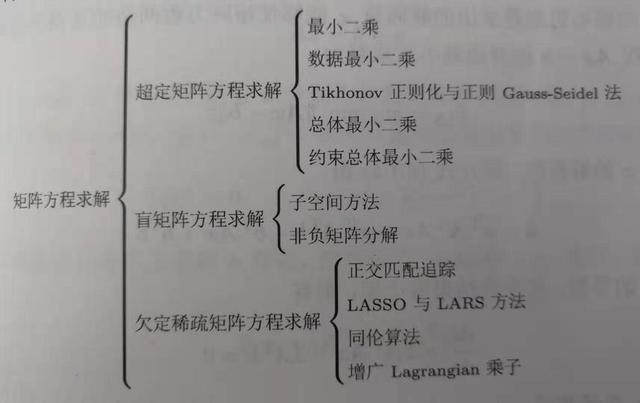## 非齐次线性方程组

$A_{m \times n} x = b_{m \times 1}$

• r(A) > r(A | b) 不可能，因为增广矩阵的秩大于等于系数矩阵的秩
• r(A) < r(A | b) 方程组无解；
• r(A) = r(A | b) = n，方程组有唯一解；
• r(A) = r(A | b) < n，方程组无穷解；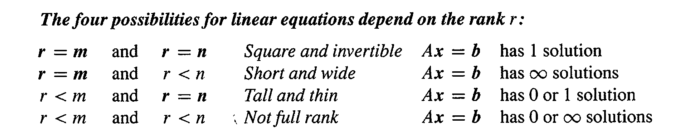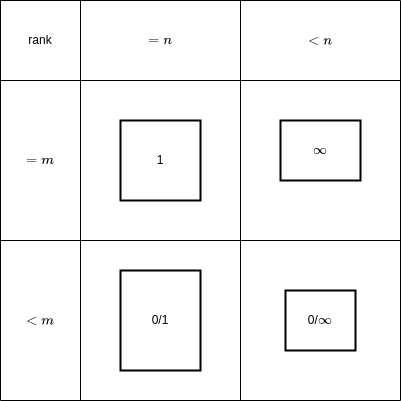### 非齐次线性方程组的最小二乘问题

$x^* = \arg \min_x{\|Ax - b\|}_2^2$

m个方程求解n个未知数，有三种情况：

• m=n
• 且A为非奇异，则有唯一解 $$x=A^{-1}b$$
• m>n，超定问题（overdetermined）
• $$x=A^{+}b$$
• m<n，欠定问题（underdetermined）

## 齐次线性方程组

$A_{m \times n} x = 0$

• r(A) = n
• A 是方阵，该方程组有唯一的零解
• A 不是方阵(m>n)，解空间只含有零向量
• r(A) < n
• 该齐次线性方程组有非零解，而且不唯一（自由度为n-r(A))

### 齐次线性方程组的最小二乘问题

$\min{\|Ax\|}_2^2 \quad s.t. \quad \|x\| = 1$

• 最小二乘解为 矩阵 $$A^TA$$ 最小特征值所对应的特征向量
• $$\text{EVD}(A^{T}A)=[V,D]$$，找最小特征值对应的V中的特征向量
• $$\text{SVD}(A)=[U,S,V]$$，找S中最小奇异值对应的V的右奇异向量

• Choleskey
• QR